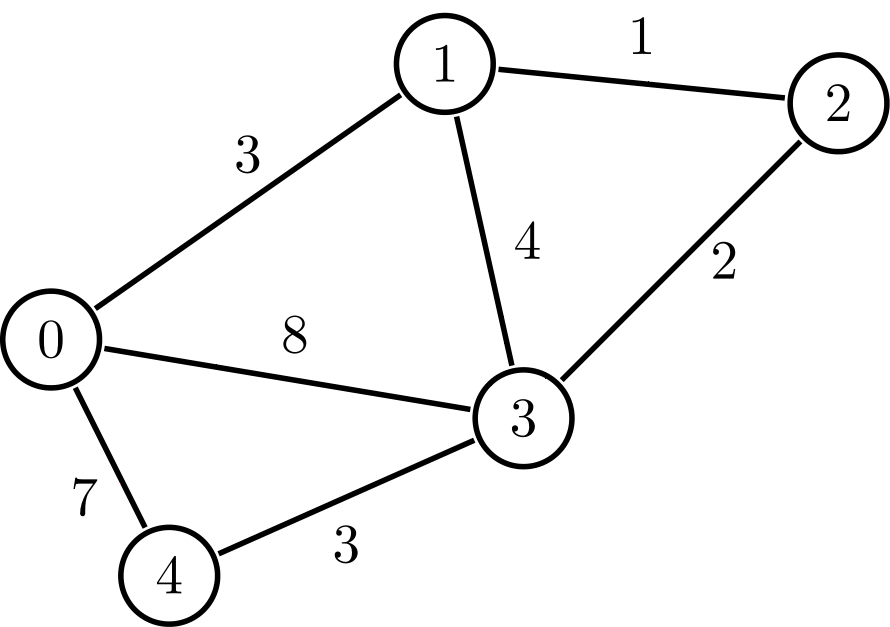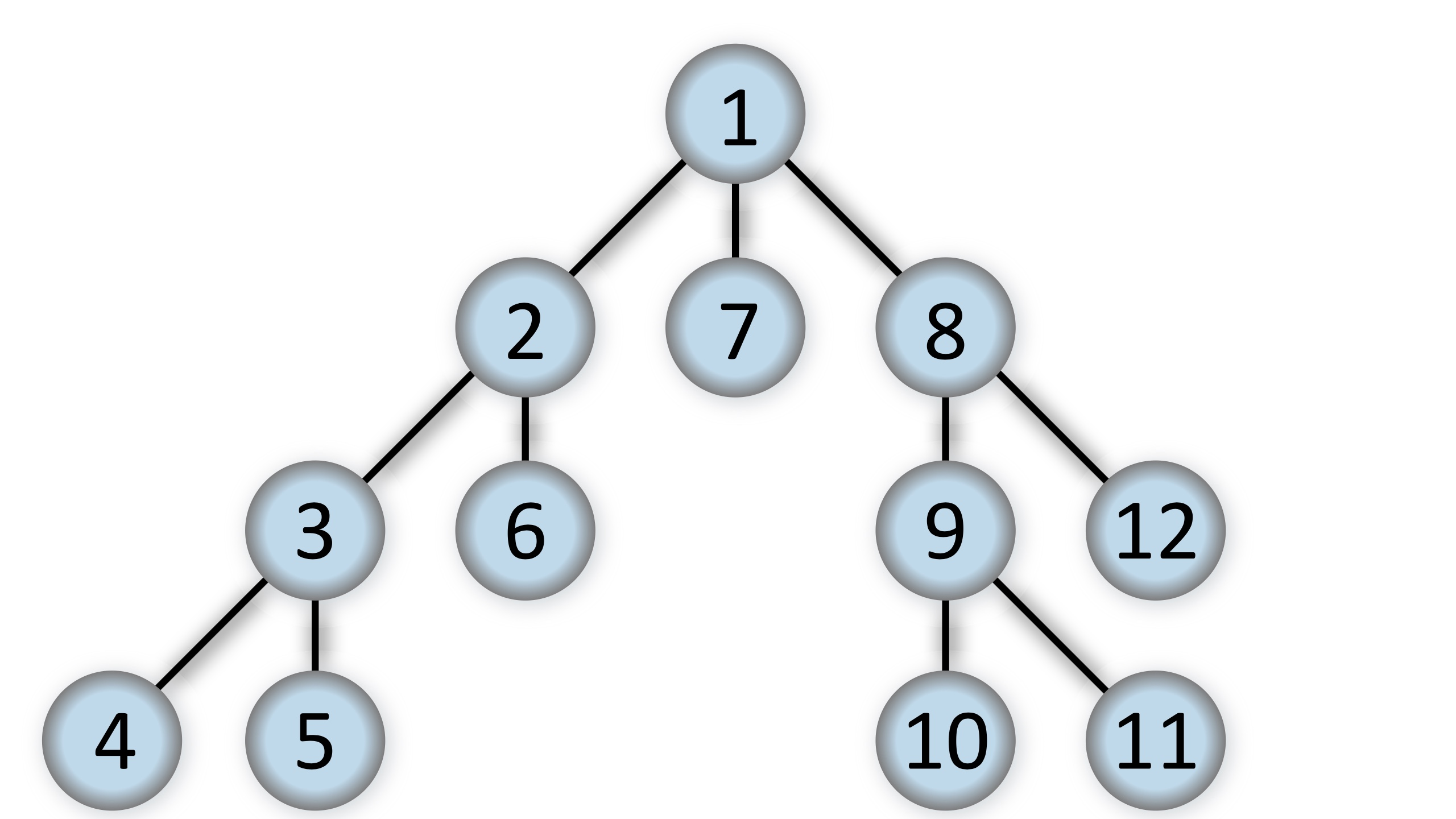# 7 Algorithms Every Programmer Should Know

ProgrammingAs a programmer, you’ve probably encountered a wide range of algorithms during the course of your career. For any programmer, becoming adept in a variety of algorithms is a must.

It’s difficult to keep track of what’s important with so many algorithms. This list will make it reasonably easy for you to prepare for an interview or simply brush up on your skills. Continue reading to learn about the most important algorithms for programmers.

#### 1. Dijkstra’s Algorithm

Edsger Dijkstra was one of the most influential computer scientists of his day, and he made significant contributions to a variety of fields in computing, including operating systems, compiler design, and much more. Dijkstra’s shortest path algorithm for graphs, often known as Dijkstra’s Shortest Path Algorithm, is one of his most important contributions.

Dijkstra’s approach locates the shortest path from a source to all graph vertices in a graph. Every time the algorithm iterates, a new vertex is added that is the shortest distance from the source and does not exist in the current shortest path. Djikstra’s algorithm makes use of this greedy feature.A set is commonly used to implement the algorithm. When using a Min Heap, Dijkstra’s approach is incredibly efficient; finding the shortest path takes only O(V+ElogV) time (V is the number of vertices and E is the number of edges in a given graph).

Dijkstra’s approach has some limits; it can only be useful on directed and undirected graphs with positive-weighted edges. The Bellman-Ford algorithm is usually preferred for negative weights.

#### 2. Merge Sort

There are a few sorting algorithms in this list, with merge sort being one of the most important. It’s a sorting algorithm that uses the Divide and Conquer programming approach to sort data efficiently. Merge sort can sort “n” numbers in O(nlogn) time in the worst-case situation. Merge sort is extremely efficient when compared to basic sorting techniques like Bubble Sort (which requires O(n2) time).The array to be sorted is periodically subdivided into subarrays until each subarray consists of a single number in merge sort. The recursive method then merges and sorts the subarrays repeatedly.

#### 3. Quicksort

Quicksort is another Divide and Conquer programming technique-based sorting algorithm. In this approach, a pivot element is initially, and then the entire array is partitioned around it.

As you may expect, a good pivot is essential for an effective sort. A random element, the media element, the first element, or even the last element can serve as the pivot.

Quicksort implementations frequently differ in how they determine a pivot. Quicksort will sort a big array with a good pivot in O(nlogn) time in most cases.

Quicksort’s general pseudocode continually partitions the array on the pivot and places it in the correct subarray position. It also positions components smaller than the pivot to the left and larger elements to the right of the pivot.

One of the first graph algorithms taught to students is Depth First Search (DFS). DFS is a fast algorithm for traversing or searching a graph. It can also tweak to work as a tree traversal tool.Starting from any arbitrary node, the DFS traversal dives into each adjacent vertex. When there are no unvisited vertex or a dead-end, the algorithm reverts. To keep track of the visited nodes, DFS commonly uses a stack and a boolean array. DFS is easy to use and extremely efficient; it operates on the principle of (V+E), where V is the number of vertices and E is the number of edges.

Topological sort, recognizing cycles in a graph, pathfinding, and finding strongly connected components are all examples of DFS traversal applications.

A level order traversal for trees is known as breadth-first search (BFS). BFS is a DFS-like algorithm that works in O(V+E). BFS, on the other hand, employs a queue rather than a stack. DFS goes deep into the graph, whereas BFS looks at it from all sides.The BFS algorithm utilizes a queue to keep track of the vertices. Unvisited adjacent vertices are visited, marked, and queued. If the vertex doesn’t have any adjacent vertice, then a vertice is removed from the queue and explored.

BFS is commonly used in peer-to-peer networks, shortest path of an unweighted graph, and to find the minimum spanning tree.

Binary Search is a straightforward approach for locating a specific member in a sorted array. It works by dividing the array in half repeatedly. The left side of the middle element is processed further if the necessary element is smaller than the middlemost element; otherwise, the right side is halved and searched again. The procedure is repeated until the required component is discovered.Binary search has an O(logn) worst-case time complexity, making it particularly efficient for scanning linear arrays.

#### 7. Minimum Spanning Tree Algorithms

A graph’s minimum spanning tree (MST) has the lowest cost of all conceivable spanning trees. A spanning tree’s cost is determined by the weight of its edges. It’s worth noting that more than one minimum spanning tree can exist. Kruskal’s and Prim’s are the two most common MST algorithms.

The MST is created via Kruskal’s algorithm, which adds the edge with the lowest cost to an expanding set. The algorithm sorts edges by weight before adding them to the MST in order of decreasing weight.

It’s worth noting that the algorithm ignores edges that create a cycle. For sparse graphs, Kruskal’s approach is preferable.

Prim’s Algorithm is also greedy and works well with dense graphs. Prim’s MST is based on the concept of having two unique sets of vertices: one that contains the growing MST and the other that contains unneeded vertices. The minimal weight edge that will connect the two sets is chosen on each iteration.